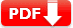# Angles Practice Test Question Answers (Elementary Geometry)

When the sum of the measures of two angles is 90°, the angles are called complementary angles. Each of them is called the complement of the other. Two angles are called adjacent angles if they have a common vertex
and a common arm but no common interior points. When the sum of the measures of two angles is 180°, the angles are called supplementary angles. Each of them is called a supplement of the other.

## Angles Practice Test

When two lines are intersected by a transversal, eight angles are formed. These angles can be classified as 4 interior angles, 4 exterior angles, 4 pairs of corresponding angles, 2 pairs of alternate interior angles, 2 pairs of alternate exterior angles, and two pairs of interior angles on the same side of the transversal.

 Test Name Angles Test Prep Type of Question Multiple Choice Question Answers Subject Math Total Question 10 Test Type Sample / Mock Test Editable & Printable PDF / Doc YES (Download link is given below) Available of Answers YES Difficulty Level Elementary Geometry

### Angles Practice Test Question Answers

#### Elementary Geometry: SET 1

1. In the following a pair of corresponding angles is

###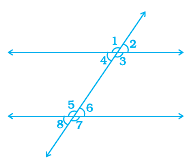🔘 A. ∠1, ∠2 🔘 B. ∠2, ∠2
🔘 C. ∠3, ∠6 🔘 D. ∠4, ∠5
🔘 E. ∠3, ∠7
 2. In the following figure AB||EF, ED||CB and ∠APE is 39°. Find ∠CQF.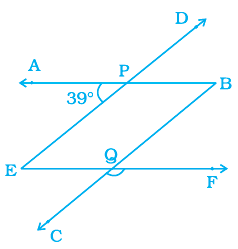🔘 A. ∠CQF= 280 🔘 B. ∠CQF= 390 🔘 C. ∠CQF= 930 🔘 D. ∠CQF= 1390 🔘 E. ∠CQF= 1410
Answer:∠CQF= 1410 (Since ED||BC and AB is a transversal, so so ∠QBP = ∠APE
[Corresponding angles] or ∠QBP = 39° Now, AB||EF and BC is a transversal. Therefore, ∠FQB = ∠QBP [Alternate interior angles] Also, ∠CQF + ∠FQB = 180° [Linear pair] ∠CQF = 180° – 39°
 3.  Out of a pair of complementary angles, one is two-third of the other. Find the angles. 🔘 A. 18° 🔘 B. 36° 🔘 C. 54° 🔘 D. 90° 🔘 E. 180°
Answer:B. 36° Let one angle be x. So, other angle = 90° – x Thus,
(2/3)× x = 90° – x or 5x = 270° or x=54° So, one angle = 54° and the other angle = 90° – 54°= 36°.
 4. In the following figure CD intersects the line AB at F, ∠ CFB = 50° and ∠ EFA = ∠ AFD. Find the measure of ∠ EFC.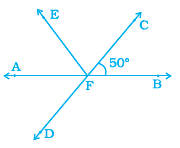🔘 A. 50° 🔘 B. 80° 🔘 C. 90° 🔘 D. 120° 🔘 E. 180°
Answer:B 80°  Solution (∠CFB = ∠AFD (Vertically opposite angles) So, x = 50° But ∠EFA = ∠AFD which gives ∠EFA = 50° Now ∠CFB + ∠EFA + ∠EFC = 180° or, 50° + 50° + ∠EFC = 180° Thus, ∠EFC = 80°. [As AB is a straight line].
 5. If the complement of an angle is 79°, then the angle will be of 🔘 A. 1° 🔘 B. 10° 🔘 C. 11° 🔘 D. 111° 🔘 E. 110°
 6. In the following figure find the value of x is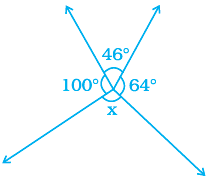🔘 A. 46° 🔘 B. 90° 🔘 C. 100° 🔘 D. 110° 🔘 E. 150°
Answer:E. 150° (Sum of all angle about a point given in the figure are equal to 360° Then, 100o + 46o + 64o + x = 360°)
 7.  In the following figure if AB || CD, ∠ APQ = 50° and ∠PRD = 130°, then ∠ QPR is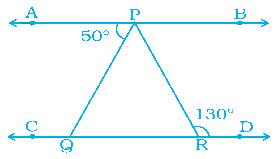🔘 A. 20° 🔘 B. 50° 🔘 C. 80° 🔘 D. 90° 🔘 E. 130°
Answer: C. 80° (Hints: ∠APQ + ∠QPR = 130° )
 8.  If angle A and angle B are supplementary and the measure of angle A is 60°, then the measure of angle B is 🔘 A. 30° 🔘 B. 60° 🔘 C. 80° 🔘 D. 90° 🔘 E. 120°
 10. In the following figure find the value of y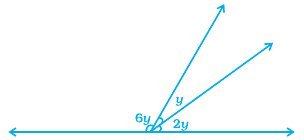🔘 A. 10° 🔘 B. 20° 🔘 C. 33° 🔘 D. 45° 🔘 E. 60°
 Document Type Download Link Free Editable Doc File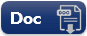Free Printable PDF File